## Example Questions

### Example Question #1 : Sequences

In the sequence 3, ____, ____, 24, what numbers can fill the two blanks so that consecutive terms differ by a common ratio?

6, 12

10, 17

12, 16

9, 18

10, 15

6, 12

Explanation:

If the common ratio is r, then the sequence can be rewritten as 3, 3r, , . We see then that , which gives us that r=2. Therefore, the missing terms are 6 and 12.

### Example Question #1 : Sequences

Find the common difference of the following sequence: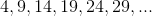Explanation:

The common difference is equal to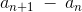Plugging our values into this equation we can find the common difference.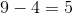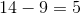Therefore, in this case the common difference is.

### Example Question #2 : Sequences

Find the common difference of the following sequence: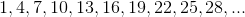Explanation:

The common difference is equal to.

Plugging in the values from this problem we get,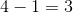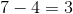Therefore, in this case the common difference is.

### Example Question #1 : How To Find The Common Difference In Sequences

Find the common difference of the following sequence: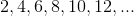Explanation:

The common difference is equal to.

Plugging in the values from this problem we get,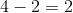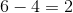Therefore, in this case the common difference is.

### Example Question #2 : How To Find The Common Difference In Sequences

The following is an arithmetic sequence. Find an explicit equation for it in terms of the common difference.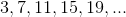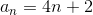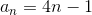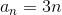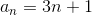Explanation:

Finding the common difference is fairly simple. We simply subtract the first term from the second. 7-3 = 4, so 4 is our common difference. So each term is going to be 4n plus something: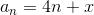We know the first term is 3, so we can plug in that to our equation.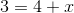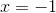So the explicit form of our arithmetic sequence is.

### All ACT Math Resources